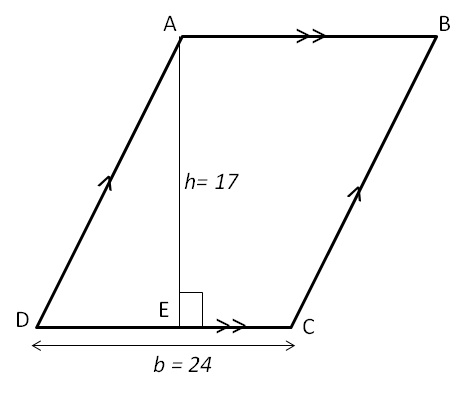Area of parallelogram

## Objective:

To show that the area of parallelogram is product of its base and height.

## Theory:

A parallelogram is a simple quadrilateral with two pairs of parallel sides.### Properties:

1. The opposite or facing sides of a parallelogram are of equal length.
2. The opposite angles of a parallelogram are of equal measure.
3. Opposite sides of a parallelogram are parallel (by definition) and so will never intersect.
4. The area of a parallelogram is twice the area of a triangle created by one of its diagonals.
5. Area of the parallelogram = base x height.

### Example:Find the area of parallelogram ABCD with base 24 m and height 17 m.

### Solution :

Area of the parallelogram = base x height
=24 X 17
=408
∴ The area of parallelogram ABCD is 408 m2